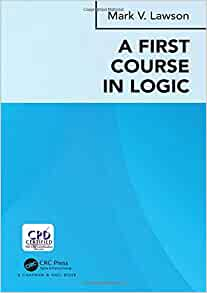A first course in logic

This is the errata page for my book
published in December 2018Name Typos Hind Zantout Examples 1.3.5(1). An extraneous negation sign has found its way into the formula in the truth table. Erase it.  Due to printer's devil. Hind Zantout Examples 1.4.15 refer to Example 4.1(1) this should be Example 1.4.4(1). Alexander Evetts and Paul Klinsberg Exercises 2.2, Question 13. The final symbol in the equation should be an x not a y. Calum Wyness Question 4 of Exercises 3.1. The notation used in the solution does not match that used in Example 3.1.4. In fact, you need to refer to Example 3.1.19. The first-order language of logic used has two 1-place predicate symbols A and B and two 2-place predicate symbols P and Q. The formulae have to be written in this language. The formulae you write down are then interpreted as the given relations in the family tree. Mahsa T Manzari Page 51. Example 1.8.8. There are two typos in the second displayed logical equivalence. In the third brackets, there should be no negation in front of the atom z. In the fourth brackets, the conjunction should be a disjunction. Jennie Hansen Page 164. I(X)[d] should read I(X_{1})[d] in both (b) and (c). Euan Aitken Solution to Exercises 1.3 Question1(a). The truth assignment leading to F is P=T, q=F and r = T (the value for r is missing). Aaron Molesbury Solution to Question 1 of Exercises 1.5. The penultimate connective in the solution should also be a conjunction. Raul Alonso Gonzalez Page 204.  Solution to part (c) of Question 3 of Exercises 1.6. The second column labelled nand should be labelled nor. Jolene Stout Question 2(l) of Exercises 3.1. The solution has an `e' where it should have a `c'.